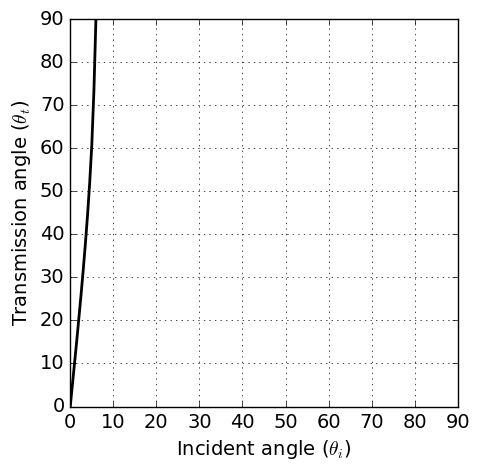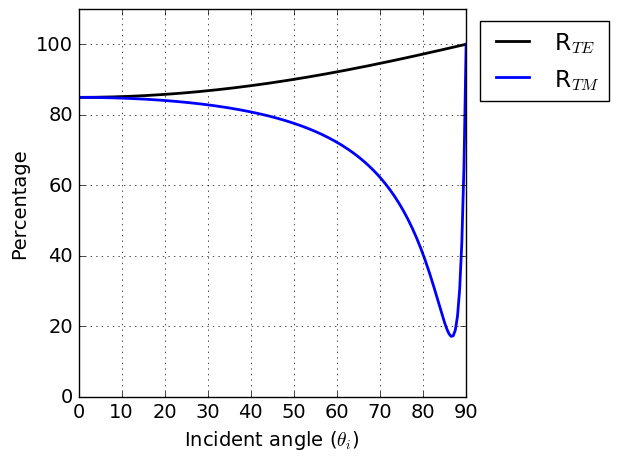# Total Reflection and Brewster’s Angle

Purpose

We first identify total reflection and brewster angle for a dielectric media, then relate them to conductive medium.

## Total Reflection

For a perfect dielectric, the conductivity is zero and the permeability is that of free space that is, $$\mu_1=\mu_2=\mu_0$$. In this case, Snell’s law reduces to:

(325)$\frac{\text{sin} \theta_i}{\text{sin} \theta_t} = \frac{k_1}{k_2} = \Big(\frac{\epsilon_2}{\epsilon_1}\Big)^{1/2} = n_{12}$

where $$n_{12}$$ is the relative index of refraction. If $$\epsilon_2 > \epsilon_1$$ then $$n_{12} > 1$$. Under this situation, for any angle of incidence $$\theta_i$$ there is a real angle of transimission $$\theta_t$$. On the other hand, if $$\epsilon_2 < \epsilon_1$$ then $$\theta_t$$ is real only when $$n_{12} \text{sin} \theta_t \leq 1$$. Total reflection occurs when $$n_{12}\text{sin} \theta_t > 1$$, and indicates that the wave cannot pass through and is entirely reflected. For the reflection from a conductive surface, a total reflection occurs when $$\sigma_1 > \sigma_2$$. Fig. 78 illustrate this.Fig. 78 Tranmission angle $$\theta_t$$ as a function of the incident angle $$\theta_i$$ when $$\sigma_1$$ = 1 S/m and $$\sigma_2$$ = 0.1 S/m. Magnetic permeability and dielectric permittivitivy assumed to be those of free-space ($$\epsilon = \epsilon_0$$ and $$\mu = \mu_0$$)

## Brewster’s Angle

From derived reflection coefficients for TE mode in Fresnel Equations, the reflection coefficient for perfect dielectric can be written as

$r_{TE} = \frac{(\epsilon_1)^{1/2} \text{cos} \theta_i - (\epsilon_2)^{1/2} \text{cos} \theta_t}{(\epsilon_1)^{1/2} \text{cos} \theta_i + (\epsilon_2)^{1/2} \text{cos} \theta_t}$

With Snell’s law of refraction shown in Eq. (325), above equation can be modified as

$r_{TE} = \frac{\text{cos} \theta_i \text {sin} \theta_t - \text{cos} \theta_t \text {sin} \theta_i}{\text{cos} \theta_i \text {sin} \theta_t + \text{cos} \theta_t \text {sin} \theta_i} = \frac{\text {sin} (\theta_t - \theta_i)}{\text {sin}(\theta_t + \theta_i)}$

Similarly, the reflection coefficient for TM mode can be obtained as

$r_{TM} = \frac{\text {tan} (\theta_t - \theta_i)}{\text {tan}(\theta_t + \theta_i)}$

Power reflection coefficient for TE and TM mode can be

$R_{TE} \equiv |r_{TE}|^2 = \frac{\text {sin}^2 (\theta_t - \theta_i)}{\text {sin}^2(\theta_t + \theta_i)}$
$R_{TM} \equiv |r_{TM}|^2 = \frac{\text {tan}^2 (\theta_t - \theta_i)}{\text {tan}^2(\theta_t + \theta_i)}$

Accordingly, power transmission coefficient will be

$T_{TE} \equiv 1-|r_{TE}|^2$
$T_{TM} \equiv 1-|r_{TM}|^2$

If $$(\theta_t + \theta_i) \rightarrow \pi/2$$, then $$\text{tan}(\theta_t + \theta_i) \rightarrow \infty$$, and $$r_{TM} \rightarrow 0$$. The reflected and refracted waves are normal to one another, and

$\text {sin} \theta_t = \text {sin} (\pi/2 - \theta_i) = \text {cos} \theta_i$

so that Eq. (325) becomes

$\text {tan} \theta_i = \Big(\frac{\epsilon_2}{\epsilon_1}\Big)^{1/2} = n_{12}$

The angle that this equation satisfies is known as the Brewster angle. The reflection from a conductive surface, there will be a minimum in $$R_{TM}$$, analogous to the Brewster angle, for some particular angle of incidence. No such minimul occurs in $$R_{TE}$$. Fig. 79 illustrate this.Fig. 79 The power reflection coefficient $$R_{TE}$$ and $$R_{TM}$$ versus angle of incidence for plane wave at air-earth interface. The conductivity and dielectric permitivity of earth are taken to be 0.01 S/m, and $$\epsilon = \epsilon_0$$, respectively. The frequency is 6 x 10 5 Hz.

## App

Fig. 78 and Fig. 79 are generated by the Reflection and Refraction app that you can adjust conductivity of each medium, and obtain corresponding transmission angle, power reflection and transmission coefficients as a function of incident angle. Below link will direct you to the app: